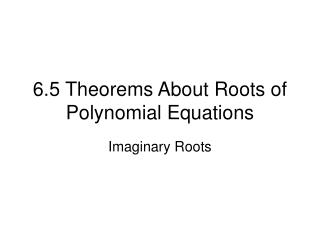DownloadDownload Presentation6.5 Theorems About Roots of Polynomial Equations

6.5 Theorems About Roots of Polynomial Equations

Télécharger la présentation6.5 Theorems About Roots of Polynomial Equations

- - - - - - - - - - - - - - - - - - - - - - - - - - - E N D - - - - - - - - - - - - - - - - - - - - - - - - - - -
Presentation Transcript

1. 6.5 Theorems About Roots of Polynomial Equations Imaginary Roots

2. POLYNOMIALS and THEOREMSTheorems of Polynomial Equations There are 4 BIG Theorems to know about Polynomials Rational Root Theorem Irrational Root Theorem Imaginary Root Theorem Descartes Rule

3. Example (Imag RT) 1. For polynomial has roots 5 and 3i 3 - 3i Degree of Polynomial ______ Other roots ______ Why not -5 ? For real numbers we do not need to worry about the conjugate. Only the complex part has a second root. Why a third degree polynomial? (x-3i)(x+3i)(x-5) 2. For polynomial has roots -5i, 4 – 2i 4 5i , 4 + 2i Other roots __________ Degree of Polynomial ______

4. Write a polynomial given the roots 4, 2i, -√5 Other roots are -2i and √5 Put in factored form y = (x – 4)(x + 2i)(x – 2i)(x + √5)(x – √5) Decide what to FOIL first

5. y = (x – 4)(x + 2i)(x – 2i) (x + √5)(x – √5) x 2i X +√5 x -2i x -√5 X2 2X i X2 X √5 -4i2 = 4 -2X i -X √5 -5 (x² +4) (x² – 5)

6. y = (x – 4)(x4 – 5x² + 4x² – 20) y = (x – 4)(x4 – x² – 20) BOX it!!! y = (x – 4)(x4 – x² – 20) x2 4 x4 -x2 -20 x2 -5 x -4 X4 4x2 X5 -X3 -20X -5X2 -4X 4 4x2 -20 80 (x4 – 5x² + 4x² – 20) y = x5 – 4x4 – x3 + 4x² – 20x + 80 y = (x4 – x² – 20)

7. Write a polynomial given the roots1 + i Note: When you box or foil complex numbers, you will not have any imaginary numbers left in the answer. If you do, go back and check your arithmetic. X –1 –i X -1 i Other root is 1 – i Put in factored form y = (x – (1 + i))(x – (1 – i)) Distribute the negatives y = (x – 1 – i )(x – 1 + i) FOIL or BOX to finish it up y = x² – 2x + 2

8. Let’s Try One • Find a fourth-degree polynomial equation with integer coefficients that has the given numbers as roots: 3 + i and -2i (Note: this is problem #29 on tonight’s homework)